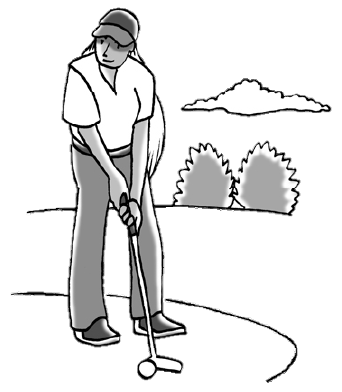### Home > CCA2 > Chapter Ch11 > Lesson 11.2.4 > Problem11-76

11-76.MINIATURE GOLF

Olivia loves playing putt-putt golf. In putt-putt golf you do not swing at a golf ball, but rather you only putt the golf ball (tap the ball with a club so that it rolls into a hole). Olivia experimented with her new club to determine whether or not she played better with it. Each time before she putted a golf ball, Olivia flipped a coin to determine whether she would use her new club or the old one. She experimented on $80$ putts. With the new club, Olivia made $25\%$ of the $40$ putts, while with the old one, she made only $15\%$ of the $40$ putts. Using the steps below, help Olivia decide whether or not her new golf club is really better, or if this difference can be explained by sample-to-sample variability.

1. What is the difference in the proportions (new club minus old club)? Express your answer as a decimal.

$25\%-15\%$

2. Olivia needs a computer simulation to determine the sample-to-sample variability:

1. Out of the $80$ putts, how many went into the hole?

$(25\%$ of $40)+(15\%$ of $40)$

2. A model of this situation needs to represent $80$ putts. What will the numbers $1$ to $16$ represent? What will the numbers $17$ to $80$ represent?

$16$ out of Olivia's $80$ putts went in the hole.

3. Conduct one simulation of $80$ putts. What proportion of the putts in your simulation went into the hole? What proportion did not go into the hole? What is the difference in the proportion (proportion that went into the hole minus proportion that did not go in)? Olivia ran the simulation $50$ times and calculated the difference in the proportion of putts that went into the hole and those that did not go into the hole for each simulation. From her results, she predicted the true difference in proportion of all her putts was $0.10\pm0.146$.

Using a graphing calculator, randlnt$(0,1,180)$. $0$ = a miss

3. What does a difference of zero mean in the context of this problem? Is a difference of zero a plausible result considering your margin of error?

The margin of error is from $0.10-0.146$ to $0.10+0.146$.
Is $0$ within the margin of error?

4. Are you convinced that there is a true difference between the new club and the old club?